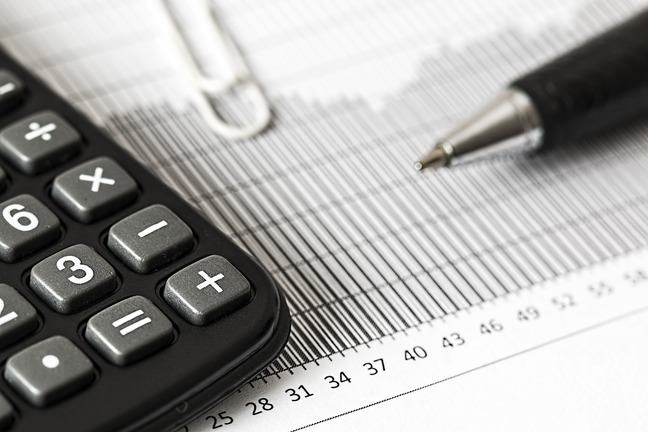2.6

## SOAS University of London# Return and risk of financial securities

Before we consider the risk of a portfolio of assets, we have to look at the risk of the individual securities which are included in the portfolio.

The risk of a security is related to the variability of its returns. Suppose we have an asset $X$, whose return $R_X$ can be equal to 10%, 6% or 2% with probability 20%, 60% and 20% (or 1/5, 3/5 and 1/5) respectively. We can summarise the return on $X$ with the following table.

Probability Return: $R_X$
0.20 10$\%$
0.60 6$\%$
0.20 2$\%$

How can we measure the variability of the returns on $X$?

First, let’s compute the expected return on $X$, indicated by $\mu_X$, or sometimes $E(R_X)$. This is a measure of the average return, and can be calculated as each possible return multiplied by the probability it will occur:

The expected return on asset $X$ is 6%. This is a measure of what investors can expect to gain by investing in the asset. However, in reality the return can be as high as 10% or as low as 2%. How can we measure the variability of the returns on the asset?

To do this, we compute the variance. We take the difference of each possible return from the mean, 6%:

Probability Return: difference from the mean $R_X - \mu_X$
0.20 10$\%$ – 6$\%$ = 4$\%$ = 0.04
0.60 6$\%$ – 6$\%$ = 0$\%$ = 0.00
0.20 2$\%$ – 6$\%$ = –4$\%$ = –0.04

We then square the differences of the returns from the mean (can you see why we need to do this step?):

Probability Return: difference from the mean, squared $(R_X - {{\mu_X}^2})$
0.20 0.0016
0.60 0
0.20 0.0016

Finally, we take an average of the squared differences from the mean, again using the probability that each return will occur. This is the variance of the returns, $\sigma_x^2$ :

The variance is a measure of the variability of the returns. An easier measure to interpret is the standard deviation, which is computed as the square root of the variance and is denoted as $\sigma_x$:

In our example, the standard deviation is:

How do we interpret the standard deviation of the returns? The larger the standard deviation, the greater the uncertainty associated with the returns on the asset.

To see how the standard deviation is related to the variability of the returns on the asset, let us consider a second security $Y$ whose possible returns $R_Y$ are shown in the following table:

Probability Return: $R_Y$
0.20 16%
0.60 6%
0.20 -4%

Compare the returns on $X$ with the returns on $Y$. You will see that when things are good for asset $Y$, they are very good (16%), but when they are bad, they are very bad (–4%). The expected return on $Y$, $\mu_Y$, or $E(R_Y)$, is computed as:

The expected return on asset $Y$ is 6%, the same as for asset $X$. On average, the two securities are expected to have the same return. However, asset $Y$ looks riskier than asset $X$, because it could generate larger profits but also losses. What will the standard deviation for $Y$ look like? Let us first compute the variance. We can use the following table:

Probability $R_Y - \mu_Y$ $(R_Y - \mu_Y)^2$
0.20 16% – 6% = 10% = 0.10 0.01
0.60 6% – 6% = 0% = 0.00 0
0.20 –4% – 6% = –10% = –0.10 0.01

The variance $\sigma_Y^2$ is:

and the standard deviation $\sigma_Y$ is:

The standard deviation of $Y$, $\sigma_Y = 6.32\%$, is larger than the standard deviation of $X$, $\sigma_X =2.53\%$. Asset $Y$ has a greater variability than asset $X$.

Can you see how the standard deviation of the returns is a measure of the risk of the asset?

Use the comments to share your views.